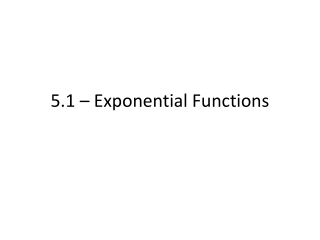DownloadDownload Presentation5.1 – Exponential Functions

# 5.1 – Exponential Functions

Télécharger la présentation## 5.1 – Exponential Functions

- - - - - - - - - - - - - - - - - - - - - - - - - - - E N D - - - - - - - - - - - - - - - - - - - - - - - - - - -
##### Presentation Transcript

1. 5.1 – Exponential Functions

2. Exponential Function = a type of function in which a constant is raised to a variable power • Many real-life applications using exponential functions • Exponential functions will be of the form : f(x) = ax

3. Behavior • To analyze the behavior of an exponential function, remember… • a-x = 1/(ax) • For any exponential function with a ≠ 1; • A function is decreasing if 0 < a < 1 • f(x) -> ∞ as x -> - ∞ • f(x) -> 0 as x -> ∞

4. Behavior continued… • A function is increasing if a > 1 • f(x) -> 0 as x -> - ∞ • f(x) -> ∞ as x -> ∞

5. Exponential Equations • An exponential equation may be written as a function in which variables are exponents • ax = ab • For us to solve them currently, we will attempt to create common bases

6. Example. Solve the exponential function 25x – 125 = 0 • Can we write 25 and 125 as some form of a common base? • Remember! Powers, not multiplication

7. Example. Solve the exponential equation 32x-1 = 27

8. Example. Solve the exponential equation 2x+1 = 643

9. Assignment • Page 384 • 19, 22, 24, 25, 27, 32, 34, 39, 41, 43